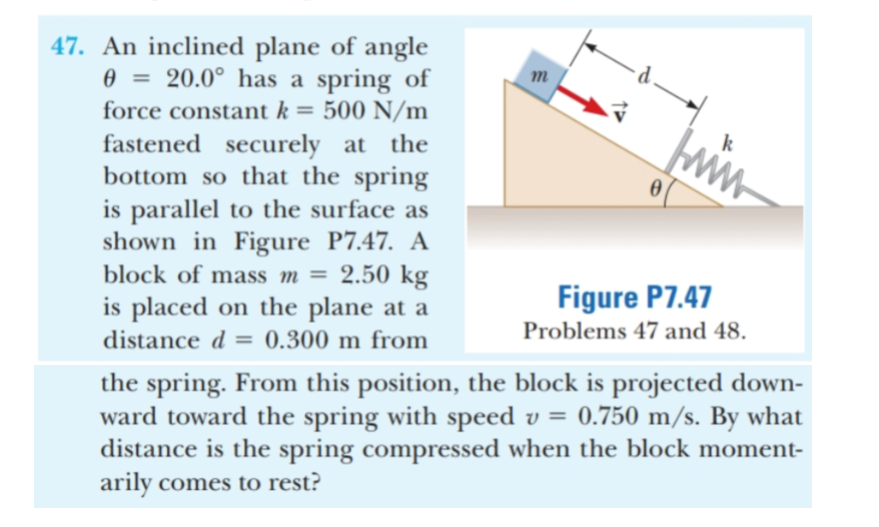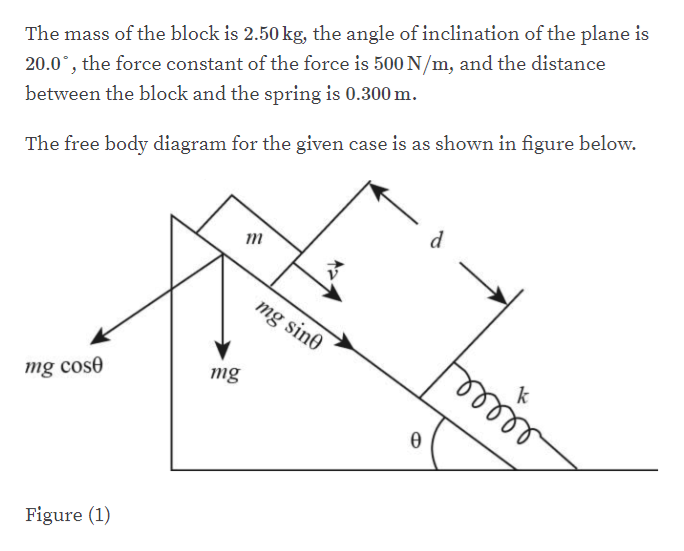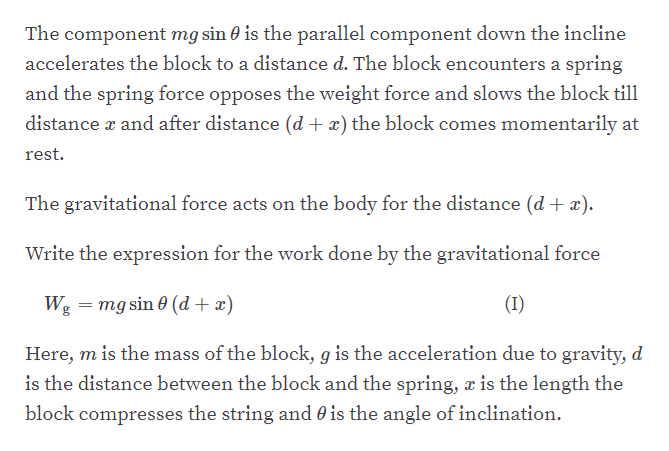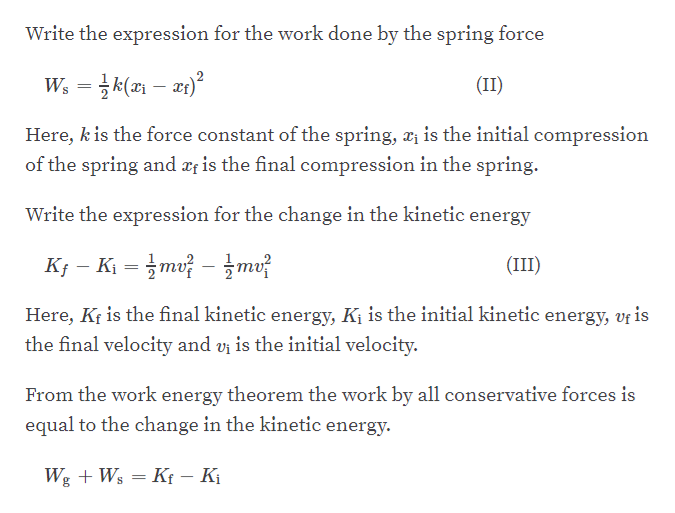# 47. An inclined plane of angle20.0° has a spring of500 N/mfastened securely at thebottom so that the springis parallel to the surface asshown in Figure P7.47. A2.50 kgis placed on the plane at amforce constant k =ww.block of mass m =Figure P7.47Problems 47 and 48distance d0.300 m fromthe spring. From this position, the block is projected down-ward toward the spring with speed v = 0.750 m/s. By whatdistance is the spring compressed when the block moment-arily comes to rest?

Question
11 viewshelp_outlineImage Transcriptionclose47. An inclined plane of angle 20.0° has a spring of 500 N/m fastened securely at the bottom so that the spring is parallel to the surface as shown in Figure P7.47. A 2.50 kg is placed on the plane at a m force constant k = ww. block of mass m = Figure P7.47 Problems 47 and 48 distance d 0.300 m from the spring. From this position, the block is projected down- ward toward the spring with speed v = 0.750 m/s. By what distance is the spring compressed when the block moment- arily comes to rest? fullscreen
check_circle

Step 1help_outlineImage TranscriptioncloseThe mass of the block is 2.50 kg, the angle of inclination of the plane is 20.0, the force constant of the force is 500 N/m, and the distance between the block and the spring is 0.300 m. The free body diagram for the given case is as shown in figure below. т mg sin0 mg cose mg Figure (1) fullscreen
Step 2help_outlineImage TranscriptioncloseThe component mg sin 0 is the parallel component down the incline accelerates the block to a distance d. The block encounters a spring and the spring force opposes the weight force and slows the block til1 distance r and after distance (d + x) the block comes momentarily at rest. The gravitational force acts on the body for the distance (d + x). Write the expression for the work done by the gravitational force Wg mg sin0 (d x) (I) Here, m is the mass of the block, g is the acceleration due to gravity, d is the distance between the block and the spring, æ is the length the block compresses the string and 0 is the angle of inclination. fullscreen
Step 3help_outlineImage TranscriptioncloseWrite the expression for the work done by the spring force (II) Here, k is the force constant of the spring, a is the initial compression of the spring and xis the final compression in the spring Write the expression for the change in the kinetic energy Kj- Ki m mv (III) Here, Kr is the final kinetic energy, Kj is the initial kinetic energy, vr is the final velocity and vi is the initial velocity. From the work energy theorem the work by all conservative forces is equal to the change in the kinetic energy. WgWs Kt - K fullscreen

### Want to see the full answer?

See Solution

#### Want to see this answer and more?

Solutions are written by subject experts who are available 24/7. Questions are typically answered within 1 hour.*

See Solution
*Response times may vary by subject and question.
Tagged in

### Other